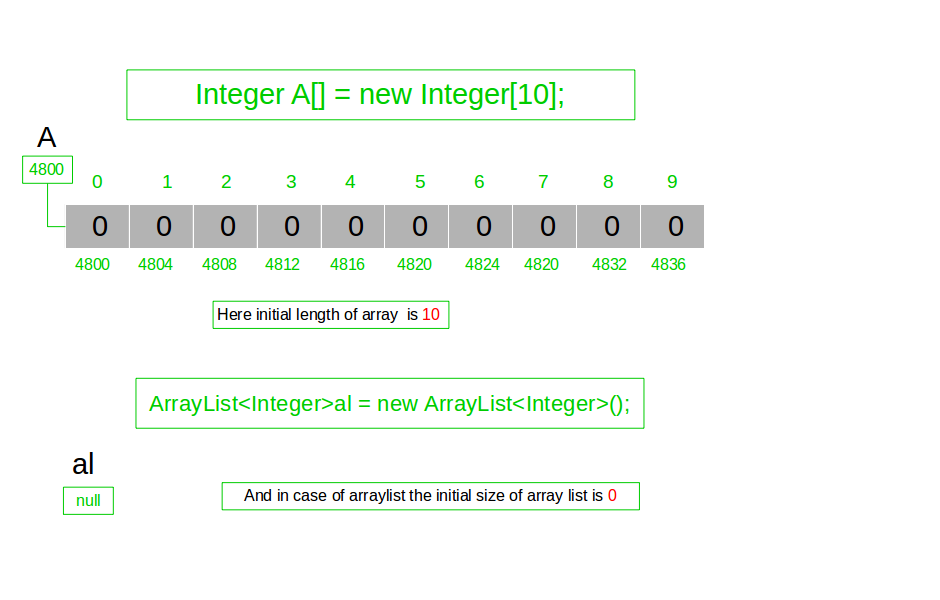# Difference between length of Array and size of ArrayList in Java

Array has length property which provides the length of the Array or Array object. It is the total space allocated in memory during the initialization of the array. Array is static so when we create an array of size n then n blocks are created of array type and JVM initializes every block by default value. Let’s see this in the following figure.On the other hand, java ArrayList does not have length property. The java ArrayList has size() method for ArrayList which provides the total number of objects available in the collection.

We use length property to find length of Array in Java and size() to find size of ArrayList.

Below is the implementation of the above idea:

 `// Java code to illustrate the difference between ` `// length in java Array and size in ArrayList ` ` `  `import` `java.util.ArrayList; ` ` `  `public` `class` `GFG { ` `    ``// main method ` `    ``public` `static` `void` `main(String[] args) ` `    ``{ ` ` `  `        ``/* creating an array A[] for 10 elements */` `        ``String A[] = ``new` `String[``10``]; ` ` `  `        ``/* store 2 elements */` `        ``A[``0``] = ``"Hello"``; ` `        ``A[``1``] = ``"Geeks!"``; ` ` `  `        ``/* print length of array A[] */` `        ``System.out.println(A.length); ``// 10 ` ` `  `        ``/* Creating an ArrayList */` `        ``ArrayList al = ``new` `ArrayList(); ` ` `  `        ``/* add 3 elements */` `        ``al.add(``"G"``); ` `        ``al.add(``"F"``); ` `        ``al.add(``"G"``); ` ` `  `        ``/* print size of ArrayList */` `        ``System.out.println(al.size()); ``// 3 ` `    ``} ` `} `

Output:

```10
3
```My Personal Notes arrow_drop_upCheck out this Author's contributed articles.

If you like GeeksforGeeks and would like to contribute, you can also write an article using contribute.geeksforgeeks.org or mail your article to contribute@geeksforgeeks.org. See your article appearing on the GeeksforGeeks main page and help other Geeks.

Please Improve this article if you find anything incorrect by clicking on the "Improve Article" button below.

Improved By : SatyaShekharBarik